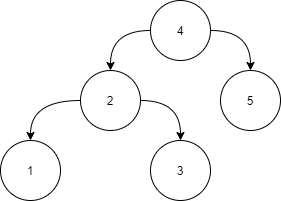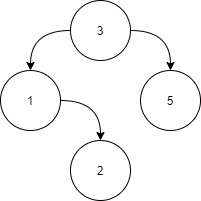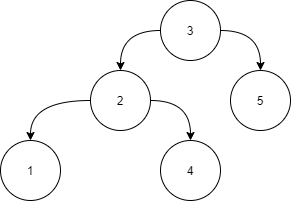4

# Validate BST

Difficulty: MEDIUM
Avg. time to solve
25 min
Success Rate
70%

Problem Statement
Suggest Edit

#### A binary search tree (BST) is a binary tree data structure which has the following properties.

``````• The left subtree of a node contains only nodes with data less than the node’s data.
• The right subtree of a node contains only nodes with data greater than the node’s data.
• Both the left and right subtrees must also be binary search trees.
``````

#### Input:``````Level 1:

All the nodes in the left subtree of 4 (2, 1, 3) are smaller
than 4, all the nodes in the right subtree of the 4 (5) are
larger than 4.

Level 2 :

For node 2:
All the nodes in the left subtree of 2 (1) are smaller than
2, all the nodes in the right subtree of the 2 (3) are larger than 2.
For node 5:
The left and right subtree for node 5 is empty.

Level 3:

For node 1:
The left and right subtree for node 1 are empty.
For node 3:
The left and right subtree for node 3 are empty.
Because all the nodes follow the property of a binary
search tree, the above tree is a binary search tree.
``````
##### Input Format:
``````The first line contains an Integer 't', which denotes the number of test cases or queries to be run. Then the test cases follow.

The first line of input contains the elements of the tree in the level order form separated by a single space.
If any node does not have a left or right child, take -1 in its place. Refer to the example below.

Example:

Elements are in the level order form. The input consists of values of nodes separated by a single space in a single line. In case a node is null, we take -1 in its place.

For example, the input for the tree depicted in the below image would be :
````````````1
2 3
4 -1 5 6
-1 7 -1 -1 -1 -1
-1 -1

Explanation :
Level 1 :
The root node of the tree is 1

Level 2 :
Left child of 1 = 2
Right child of 1 = 3

Level 3 :
Left child of 2 = 4
Right child of 2 = null (-1)
Left child of 3 = 5
Right child of 3 = 6

Level 4 :
Left child of 4 = null (-1)
Right child of 4 = 7
Left child of 5 = null (-1)
Right child of 5 = null (-1)
Left child of 6 = null (-1)
Right child of 6 = null (-1)

Level 5 :
Left child of 7 = null (-1)
Right child of 7 = null (-1)

The first not-null node (of the previous level) is treated as the parent of the first two nodes of the current level. The second not-null node (of the previous level) is treated as the parent node for the next two nodes of the current level and so on.

The input ends when all nodes at the last level are null (-1).
``````
##### Note :
``````The above format was just to provide clarity on how the input is formed for a given tree.

The sequence will be put together in a single line separated by a single space. Hence, for the above-depicted tree, the input will be given as:

1 2 3 4 -1 5 6 -1 7 -1 -1 -1 -1 -1 -1
``````
##### Output Format :
``````For each test case, return true if the binary tree is a BST, else return false.

Output for every test case will be denoted in a separate line.
``````
##### Note:
``````You are not required to print anything explicitly. It has already been taken care of. Just implement the function.
``````
##### Constraint :
``````1 <= T <= 100
1 <= N <= 1000
-10^6 <= data <= 10^6
where N is the number of nodes in the tree, T represents the number of test cases, and data denotes data contained in the node of the binary tree.

Duplicate elements can be in the right subtree or left subtree.

Time Limit: 1 sec
``````
##### Sample Input 1:
``````2
3 1 5 -1 2 -1 -1 -1 -1
3 2 5 1 4 -1 -1 -1 -1 -1 -1
``````
##### Sample Output 1:
`````` true
false
``````
##### Explanation of the Sample Input1:
``````Here we have 2 test cases, hence there are 2 binary trees

Test Case 1:
```````````` Level 1:
For node 3 all the nodes in the left subtree (1,2) are
less than 3 and all the nodes in the right subtree (5)
are greater than 3.

Level 2:
For node 1:
The left subtree is empty and all the nodes in the right
subtree (2) are greater than 1.
For node 5:
Both right and left subtrees are empty.

Level 3:
For node 2, both right and left subtrees are empty.
Because all the nodes follow the property of a binary
search tree, the function should return true.

Test Case 2:
````````````For the root node, all the nodes in the right subtree (5) are greater than 3. But node with data 4 in the left subtree of node 3 is greater than 3, this does not satisfy the condition for the binary search tree. Hence, the function should return false.
``````Console